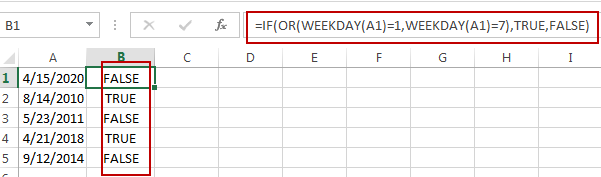# Determine If a Date Falls on the Weekend

This post will guide you how to determine if a date falls on the weekend in Excel. How do I check if a date falls on the weekend with a formula in Excel. How to check if a date is a weekend in Excel.

## Check if a Date Falls on the Weekend

Assuming that you have a list of data that contain dates in range A1:A5, and you want to check each date if it falls on the weekend. How to achieve it. You can create a formula based on the IF function, the OR function and the WEEKDAY function. Just like this:

`=IF(OR(WEEKDAY(A1)=1,WEEKDAY(A1)=7),TRUE,FALSE)`

Type this formula into Cell B1, and press Enter key in your keyboard, and then drag the AutoFill Handle from Cell B1 to B5.This formula will check if a date falls on the weekend, it will return TRUE, otherwise, it will return FALSE.

### Related Functions

• Excel IF function
The Excel IF function perform a logical test to return one value if the condition is TRUE and return another value if the condition is FALSE. The IF function is a build-in function in Microsoft Excel and it is categorized as a Logical Function.The syntax of the IF function is as below:= IF (condition, [true_value], [false_value])….
• Excel WEEKDAY function
The Excel WEEKDAY function returns a integer value representing the day fo the week for a given Excel date and the value is range from 1 to 7.The syntax of the WEEKDAY function is as below:=WEEKDAY (serial_number,[return_type])…
• Excel OR Function
The Excel OR function used to test multiple conditions and returns TRUE if any of the conditions are TRUE.The syntax of the OR function is as below:=OR(logical1, [logical2], …)…

Related Posts

If Value is Greater Than A Certain Value

IF function is frequently used in Excel worksheet to return you expect “true value” or “false value” based on the logical test result. If you want to see if a value in one cell is greater than a specific value, ...

If Cell is Not Blank

IF function is frequently used in Excel worksheet to return you expect “true value” or “false value” based on the result of created logical test. If you want to see if a cell is blank or not, and leave some ...

If Cell is Blank

IF function is frequently used in Excel worksheet to return you expect “true value” or “false value” based on the result of created logical test. If you want to see if a cell is blank or not, and leave some ...

If Cell Equals Certain Text String

IF function is frequently used in Excel worksheet to return you expect “true value” or “false value” based on the result of created logical test. If you want to see if cell equals a certain text string like “Win”, you ...

If Cell Contains Either Text1 or Text2

IF function is frequently used in Excel worksheet to return “true value” or “false value” based on the logical test result. If you want to see if cell contains certain substring1 like “abc” or substring2 like “def”, and returns true ...

If Cell Contains Certain Text OR Equals Certain Text

IF cell equals certain text IF function is frequently used in Excel worksheet to return “true value” or “false value” based on the logical test result. If you want to test values to see if they equal certain text like ...

Fix #N/A Error For VLOOKUP From Another Sheet

This post will show you how to fix the #N/A error why it occurs when you extract values from another sheet using VLOOKUP function in Excel 2016,2013,2010 or other Excel versions. How can you correct a #N/A error in VLOOKUP ...

How to Average Only Positive or Negative Numbers of a Range

Suppose both positive numbers and negative numbers exist in a table. If we want to know the average of only positive numbers in this table, we can create a formula to get average of all positive numbers with all negative ...

How to Count Dates by Day of Week in Excel

This post will guide you how to count days of week in the range of dates using a formula in Excel 2013/2016 or Excel office 365. How do I count dates by day of week in Excel. Count Dates by ...

How to Sum Data by Weekday with Different Formulas/Functions in Excel

To generate a business summary report for further investigation, we can sum income or profits for a date like Monday, Tuesday …etc. to check on which date we have the top income in a week, then we can estimate the ...

Sidebar